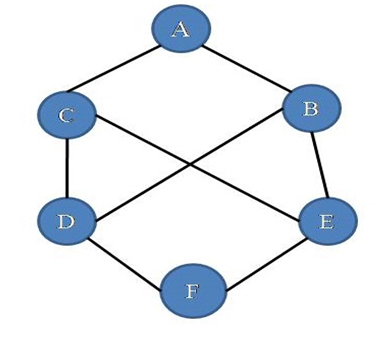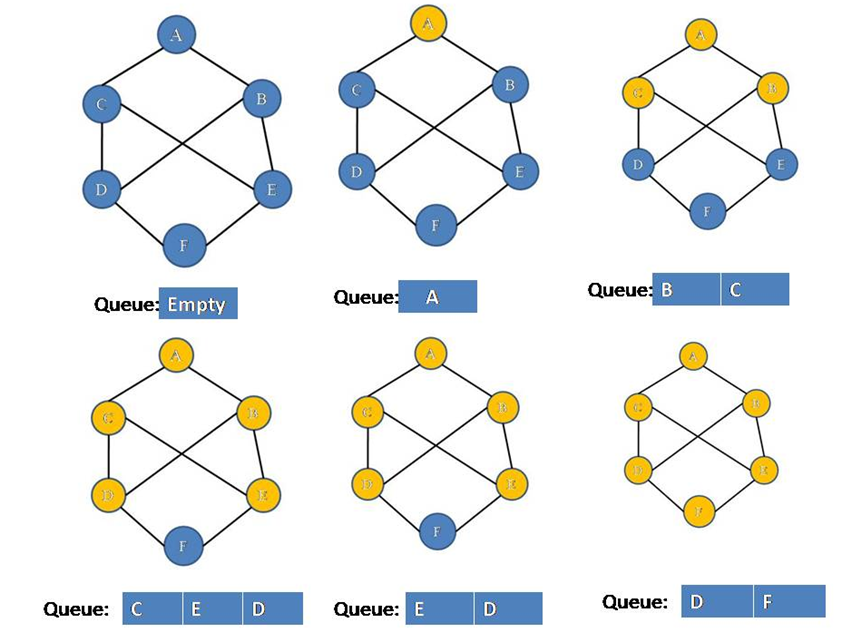# Breadth First Search (BFS) of a Graph

Submitted by Souvik Saha, on March 19, 2019

What you will learn?

How to implement Breath first search of a graph?

Breadth First Search is a level-wise vertex traversal process. Like a tree all the graphs have vertex but graphs have cycle so in searching to avoid the coming of the same vertex we prefer BFS

Algorithm:

To implement the BFS we use queue and array data structure.

There are two cases in the algorithm:

1. Whenever we visit a vertex we mark it visited and push its adjacent non-visited vertices into the queue and pop the current vertex from the queue.
2. If all the adjacent vertices are visited then only pop the current vertex from the queue.

Consider this graph,According to our algorithm, the traversal continues like,Hence all the vertices are visited then only pop operation is performed and queue will be empty finally.

C++ Implementation:

```#include <bits/stdc++.h>
using namespace std;

//	Make a pair between vertex x and vertex y
ls[x].push_back(y);
ls[y].push_back(x);
return;
}

//Breath First Search of a Graph
void BFS(list<int>*ls,int num,int x){
bool *visit= new bool[num];
for(int i=0;i<num;i++){
visit[i]=false;
}
queue<int> q;
q.push(x);
while(!q.empty()){
int s=q.front();
q.pop();
if(!visit[s]){
visit[s]=true;
cout<<s<<" ";
list<int>::iterator it;
for(it=ls[s].begin();it!=ls[s].end();it++){
q.push(*it);
}
}
}
}

void print(list<int> *ls,int num){
list<int>::iterator it;
for(int i=0;i<6;i++){
cout<<i<<"-->";
for(it=ls[i].begin();it!=ls[i].end();it++){
cout<<*it<<"-->";
}
cout<<endl;
}
}

int main(){
int num=6;

cout<<"Enter the no. of vertices : 6\n";
list<int> *ls=new list<int>[num];

print(ls,6);
cout<<"BFS"<<endl;
BFS(ls,6,0);

return 0;
}
```

Output

```Enter the no. of vertices : 6
0-->2-->3
1-->4
2-->0-->3-->5
3-->2-->4-->0
4-->3-->5-->1
5-->4-->2
BFS:
0 2 3 5 4 1
```